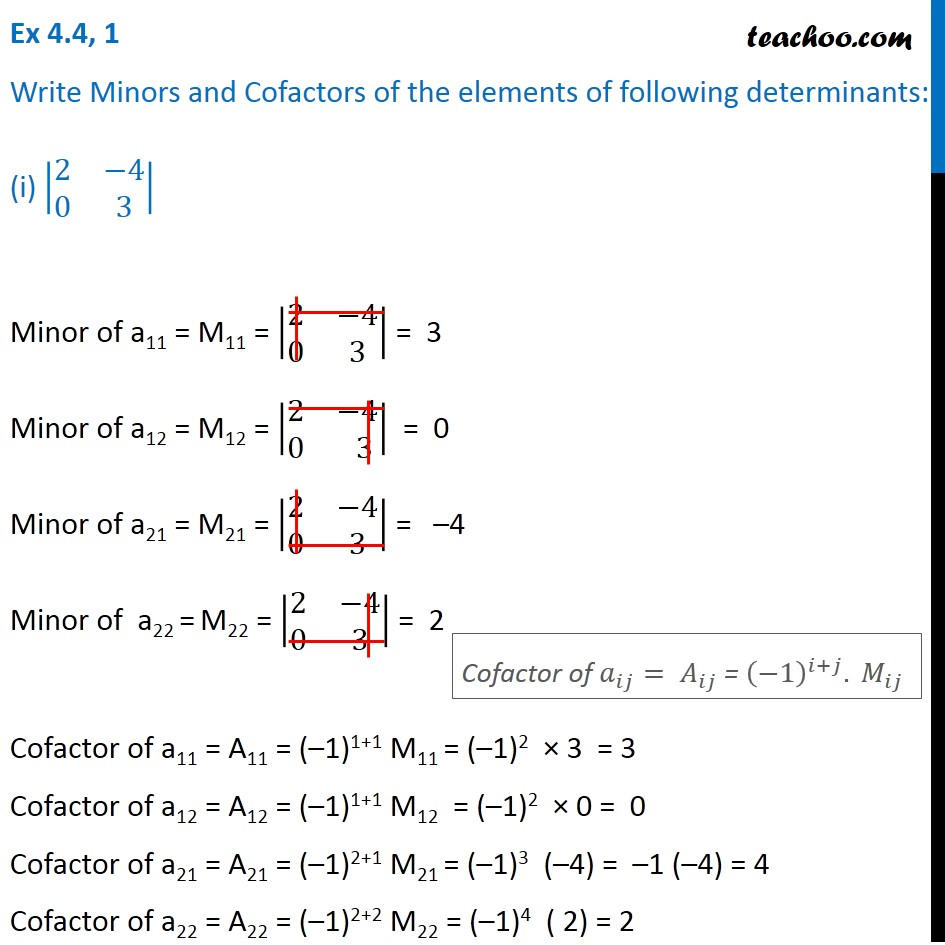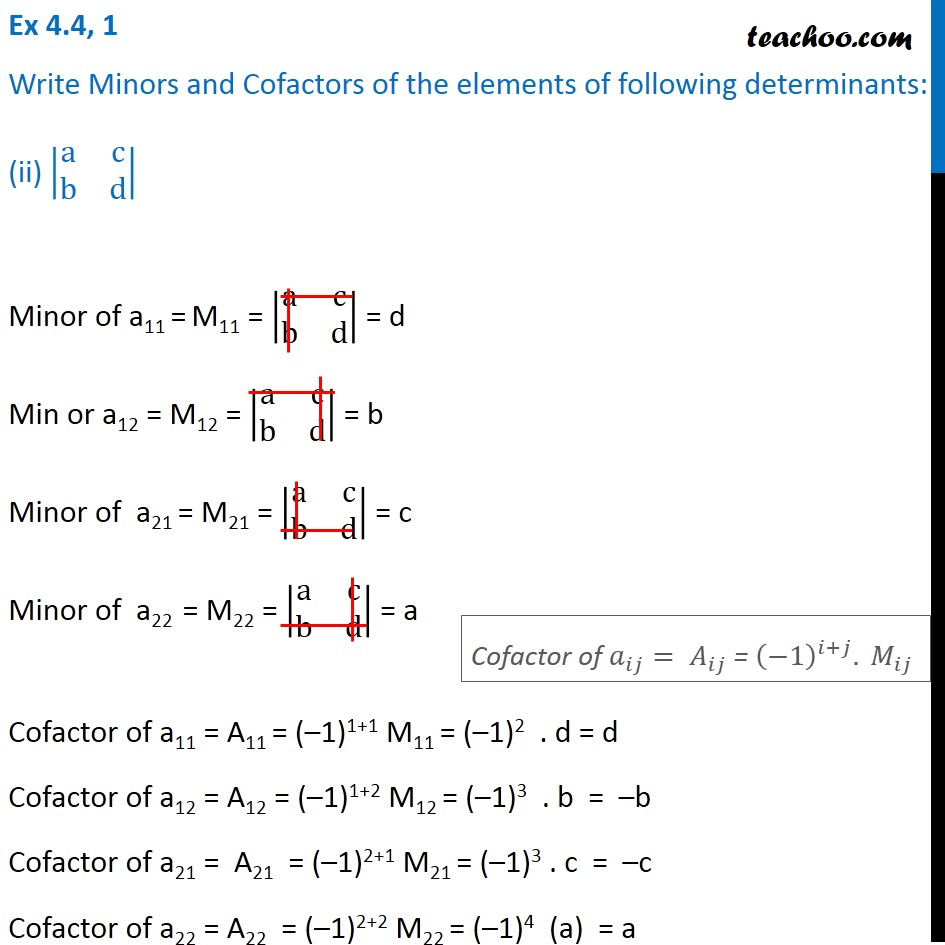Subscribe to our Youtube Channel - https://you.tube/teachoo

1. Chapter 4 Class 12 Determinants
2. Serial order wise
3. Ex 4.4

Transcript

Ex 4.4, 1 Write Minors and Cofactors of the elements of following determinants: (i) |■8(2&−4@0&3)| Minor of a11 = M11 = |■8(2&−4@0&3)| = 3 Minor of a12 = M12 = |■8(2&−4@0& 3)| = 0 Minor of a21 = M21 = |■8(2&−4@0&3)| = –4 Minor of a22 = M22 = |■8(2&−4@0&3)| = 2 Cofactor of a11 = A11 = (–1)1+1 M11 = (–1)2 × 3 = 3 Cofactor of a12 = A12 = (–1)1+1 M12 = (–1)2 × 0 = 0 Cofactor of a21 = A21 = (–1)2+1 M21 = (–1)3 (–4) = –1 (–4) = 4 Cofactor of a22 = A22 = (–1)2+2 M22 = (–1)4 ( 2) = 2 Cofactor of 𝑎_𝑖𝑗= 𝐴_𝑖𝑗 = (−1)^(𝑖+𝑗). 𝑀_𝑖𝑗 Ex 4.4, 1 Write Minors and Cofactors of the elements of following determinants: (ii) |■8(a&c@b&d)| Minor of a11 = M11 = |■8(a&c@b&d)| = d Min or a12 = M12 = |■8(a&c@b&d)| = b Minor of a21 = M21 = |■8(a&c@b&d)| = c Minor of a22 = M22 = |■8(a&c@b&d)| = a Cofactor of a11 = A11 = (–1)1+1 M11 = (–1)2 . d = d Cofactor of a12 = A12 = (–1)1+2 M12 = (–1)3 . b = –b Cofactor of a21 = A21 = (–1)2+1 M21 = (–1)3 . c = –c Cofactor of a22 = A22 = (–1)2+2 M22 = (–1)4 (a) = a Cofactor of 𝑎_𝑖𝑗= 𝐴_𝑖𝑗 = (−1)^(𝑖+𝑗). 𝑀_𝑖𝑗

Ex 4.4